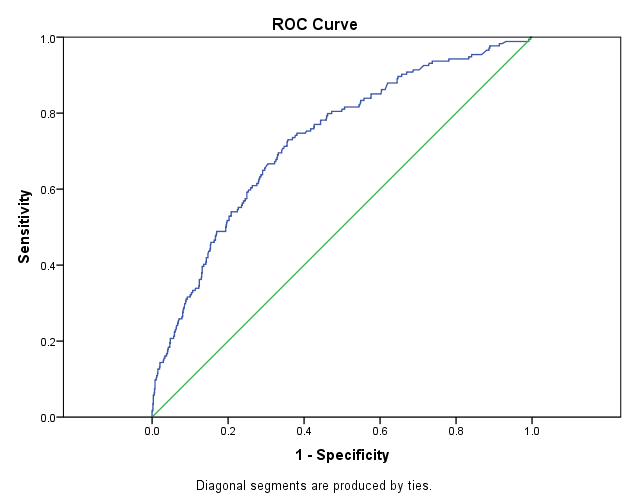# Model Metrics

Published:

This post describes model evaluation metrics used in Classification and Regression problems.

## Classification Metrics

Errors:

• Type-1: False Positive
• critical for spam detection
• Type-2: False Negative
• critical for medical diagnosis

### Precision

Correct positive from all positive results (whther true or false)

$Precision = \frac{TP}{TP+FP}$

Precision is all about positive prediction. It should be positive if model says positive. Thus, for Spam Detection, we need high precision model

### Recall, Sensitivity, Hit Rate, or True Positive Rate (TPR)

Correct positive from all actual positive (true positive and false negative)

$Recall = \frac{TP}{P} = \frac{TP}{TP+FN}$

Recall is all about ability of the model to find all relevant instances. How many positive (TP) detected from total actual positive (TP+FN). For Medical School, we need high recall model

### Combining Precision and Recall

• In a medical school, we may have different needs based on the stage of examination e.g. in pre-examination with follow-up examination.
• In case cost is high for follow-up examination, we need High Precision Model
• In case cost is low for follow-up examination, we need Low Precision Model but high Recall or Sensitivity model.

### F1 Score

• Harmonic Mean of Precision and Recall. Harmonic Mean is better since it punishes extreme values.
• HM is reciprocal of the arithmetic mean of the reciprocals.

$F1 = 2 * \frac{Precision * Recall}{Precision + Recall}$

### Fbeta Score

• F1 gives equal weight to Precision and Recall. Thus, a general formula that Recall is considered β times as important as Precision is:

$F_\beta = (1+\beta^2) \frac{Precision * Recall}{\beta^2*Precision + Recall}$

• $\beta$ equal to 1 gives equal weight so Fbeta score = F1 Score
• $\beta$ equal to 0 give F0 = Precision
• As $\beta$ increase, the score gives more value to Recall Thus
• $0 \lt \beta \lt 1$ => Precision
• $\beta \gt 1$ => Recall

High Recall Model gives most relevant results High Precision Model gives less irrelevant results

### Understanding Medical Tests

Sensitivity and specificity are widely used in medicine.

Sensitivity, Recall, Hit Rate, or True Positive Rate (TPR)

• Sensitivity is the test’s ability to correctly detect ill patients who have the condition.
• It measures proportion of sick people who are correctly identified as having the condition
• $Sensitivity = \frac{TP}{P} = \frac{TP}{TP+FN}$

Specificity, Selectivity or True Negative Rate (TNR)

• It measures proportion of healthy patients who are correctly identified as not having the condition
• $Specificity = \frac{TN}{N} = \frac{TN}{TN+FP}$

ROC dates back to 1940 where ROCs were used to measure how well a sonar signal could be detected from a noise. Now, ROC curves are used to see as how a model can distinguish true positives and true negatives.

We want a model that predict positive as positive and negative as negative.

Ideally, we want model to have high sensitivity and high specificity. However, there is a tradeoff, every model needs to pick a threshold to predict positive.

Lowering the threshold will increase Sensitivity, since $Sensitivity = \frac{TP}{P}$.

Model with threshold value 0 will predict all cases as positive, so the model predicted all positive cases as positive thus Sensitivity will be equal to 1. Similarly, if the threshold is very high say 1, then Sensitivity will be 0 since none positive case predicted as positive.

Opposite will happen with Specificity. Lowering threshold will decrease Specificity, since $Specificity = \frac{TN}{N}$

Model with threshold 0 will predict all cases as positive so TN will be 0 thus Specificity will be zero. Similarly, threshold value of 1 will make all cases as negative, so Specificity will be 1.

#### $1 - Specificity$

Specificity is predicting a real negative as negative. $1-Specificity$ will be the probability of predicting real negative as positive.

Thus, what we want is model should detect all real positive as positive (High Sensitivity) and
low number of positive who are real neagtive (Low 1-Specificity)

Best Model:
High Sensitivity => Predicts most true positives as Positive
Low on 1-specificity => few true negatives will be a positive

ROC Curve plots $1-Specificity$ (False Positive Rate) vs Sensitivity (True Positive Rate) for every possible threshold value.(credit: www.theanalysisfactor.com)

• Model predicting at chance will have ROC curve like diagonal green line
• Better model will have the curve far from diagonal line

### Area Under the Curve (AUC)

How well a model predicts i.e. how much a model is capable of distinguishing between classes

• Higher the AUC of model, better is the model

## Regression Metrics

### Mean Absolute Error (MAE)

$MAE = \frac{1}{n} \sum \mid y - \hat{y} \mid$

Since MAE is not a differentiable function, so Gradient Descent cannot be used.

$f(x) = \mid x \mid$ is not differentiable at $x=0$, since

$f^\prime(0) = \lim_{h \to 0} \frac{\mid 0+h \mid - \mid 0 \mid}{h} = \lim_{h \to 0} \frac{\mid h \mid}{h}$

LHL = -1 and RHL = 1

A function is differentaible when we zoom in it and it looks like stratight line.

Thus, we use MSE

### Mean Squared Error (MSE)

$MSE = \frac{1}{n} \sum (\mid y - \hat{y} \mid)^2$

### R2 Score

R2 score compares the model with simplest model to check if this simple model has a larger error then a linear regression model.

$R2~Score = 1 - \frac{Error_{regressionModel}}{Error_{simpleModel}} = 1 - \frac{E_1}{E_2}$

If model is good then $when~E1 < E2 \implies \frac{E_1}{E_2} \rightarrow 0 \implies R2 \rightarrow 1$

If model is bad then $when~E1 > E2 \implies \frac{E_1}{E_2} \rightarrow 1 \implies R2 \rightarrow 0$

R2 score may be negative also when the model can be arbitrarily worse.

Tags: